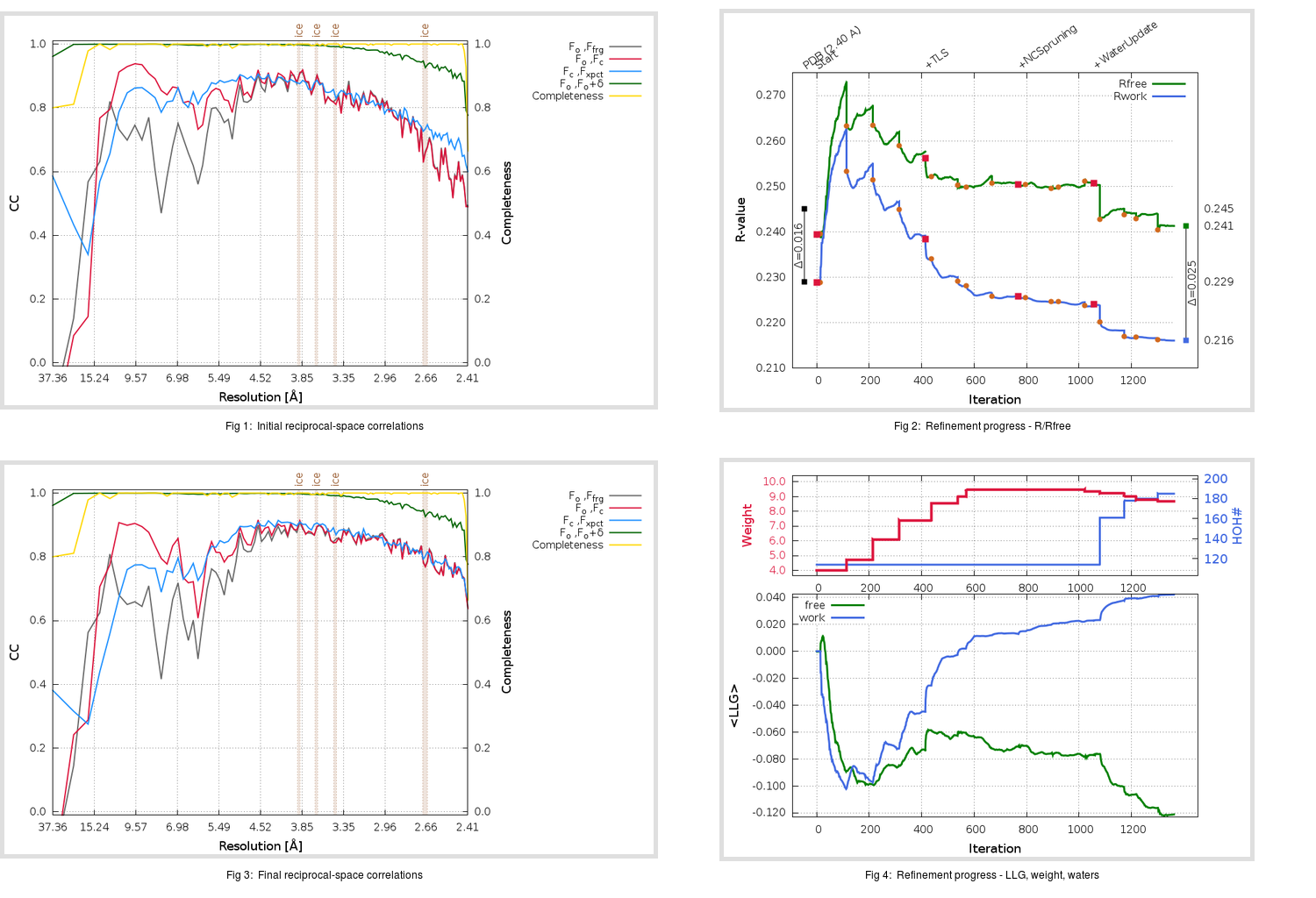Content:

```    Diffraction limits & principal axes of ellipsoid fitted to diffraction cut-off surface:
2.355         0.7291   0.0000  -0.6845       0.543 a* - 0.840 c*
2.356         0.0000   1.0000   0.0000       b*
2.340         0.6845   0.0000   0.7291       0.656 a* + 0.754 c*
```

## Deposited

` `
 Date deposited Date data collection Resolution R, Rfree 20030814 20030716 2.40 0.2290 0.2450

Molprobity (CCP4 7.0 version) summary:

```Ramachandran outliers =   2.34 %
favored =  88.48 %
Rotamer outliers      =   6.95 %
C-beta deviations     =     0
Clashscore            =  21.31
RMS(bonds)            =   0.0120
RMS(angles)           =   1.60
MolProbity score      =   3.05
Resolution            =   2.40
R-work                =   0.2290
R-free                =   0.2450
```

```Number of waters      =   114

<B> (all atoms) =   33.26 ( sd =   13.57 ) for       4783 non-hydrogen atoms
<B>   (protein) =   33.23 ( sd =   13.63 ) for       4669 non-hydrogen atoms
<B>     (water) =   34.25 ( sd =   10.75 ) for        114 non-hydrogen atoms
<B>    (others) =    0.00 ( sd =    0.00 ) for          0 non-hydrogen atoms

B min/max       (all non-hydrogen atoms) =    7.27 /   87.24
B min/max   (protein non-hydrogen atoms) =    7.27 /   87.24
B min/max     (water non-hydrogen atoms) =   10.91 /   64.01
B min/max     (other non-hydrogen atoms) =    0.00 /    0.00
```

## BUSTER (re-)refinement

` `

Molprobity (CCP4 7.0 version) summary:

```Ramachandran outliers =   0.67 %
favored =  96.33 %
Rotamer outliers      =   6.76 %
C-beta deviations     =     3
Clashscore            =   3.79
RMS(bonds)            =   0.0120
RMS(angles)           =   1.64
MolProbity score      =   2.04
Resolution            =   2.40
R-work                =   0.2160
R-free                =   0.2413
```

```Number of waters      =   185

<B> (all atoms) =   37.10 ( sd =   13.70 ) for       4854 non-hydrogen atoms
<B>   (protein) =   37.02 ( sd =   13.75 ) for       4669 non-hydrogen atoms
<B>     (water) =   39.17 ( sd =   12.29 ) for        185 non-hydrogen atoms
<B>    (others) =    0.00 ( sd =    0.00 ) for          0 non-hydrogen atoms

B min/max       (all non-hydrogen atoms) =    6.56 /   99.79
B min/max   (protein non-hydrogen atoms) =   13.48 /   99.79
B min/max     (water non-hydrogen atoms) =    6.56 /   79.11
B min/max     (other non-hydrogen atoms) =    0.00 /    0.00
```

Refinement progression:Results:

` `
 File Remark 1UK3_aB_refine.01_04_refine.pdb.gz exact refinement commands are in header 1UK3_aB_refine.01_04_refine.mtz.gz including original deposited data and several re-refinement map coefficients 1UK3_aB_refine.01_04_BUSTER_model.cif.gz including any non-standard compound restraints 1UK3_aB_refine.01_04_BUSTER_refln.cif.gz